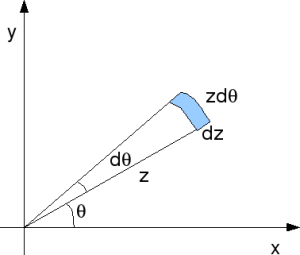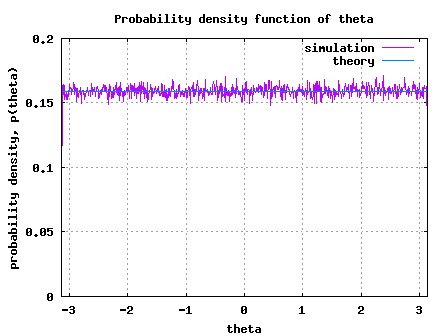- DSP log - http://www.dsplog.com -

Deriving PDF of Rayleigh random variable

Posted By Krishna Sankar On July 17, 2008 @ 5:51 am In DSP | 9 Comments

In the post on Rayleigh channel model , we stated that a circularly symmetric random variable is of the form , where real and imaginary parts are zero mean independent and identically distributed (iid) Gaussian random variables. The magnitude which has the probability density,

is called a Rayleigh random variable. Further, the phase is uniformly distributed from . In this post we will try to derive the expression for probability density function (PDF) for and .

The text provided in Section 5.4.5 of [ELECTRONIC-COMMUNICATION:PRADIP]  is used as reference.

## Joint probability

The probability density function of is

.

Similarly probability density function of is

.

As and are independent random variables, the joint probability  is the product of the individual probability, i.e,

.

The joint probability that the random variable lies between and and the random variable lies between and is,

.

## Conversion to polar co-ordinate

Given that is in the Cartesian co-ordinate form , we can convert that into the polar co-ordinate  where,
and
.Figure: Cartesian co-ordinate to polar co-ordinate

The area is Cartesian co-ordinate form is equal to the area in the polar co-ordinate form.

.

Simplifying,
.

Summarizing the joint probability density function,

.

Since and are independent, the individual probability density functions are,
,

.

## Simulation Model

Simple Matlab/Octave simulation model is provided for plotting the probability density of and . The script performs the following:

(a) Generate two independent zero mean, unit variance Gaussian random variables

(b) Using the hist() function compute the simulated probability density for both and

(c) Using the knowledge of the equation (which we just derived), compute the theoretical probability
density function (PDF)

(d) Plot the simulated and theoretical probability density functions (PDF) and show that they are in good agreement.Figure: Simulated/theoretical PDF of Rayleigh random variableFigure: Simulated/theoretical PDF of uniformly distributed theta random variable

## Reference

URL to article: http://www.dsplog.com/2008/07/17/derive-pdf-rayleigh-random-variable/

URLs in this post:

 Rayleigh channel model: http://www.dsplog.com/2008/07/14/rayleigh-multipath-channel/